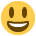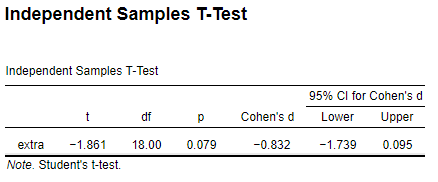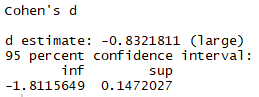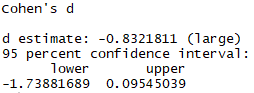#### Howdy, Stranger!

It looks like you're new here. If you want to get involved, click one of these buttons!

Supported by

# What denominator does the Cohen's d use on JASP??

As I'm getting different results when calculating Cohen's d with SD Pooled as the denominator to the results JASP is giving me.
I just want to make sure I'm reporting it correctly in my write up.

Cheers,

• I'll ask Johnny what's up. I think the issue came up before. Do you have a concrete example?
Cheers,
E.J.

• Here's a couple of examples with the JASP d and the SD Pooled d (calculated using Lee Becker's website):
M1 = 66.5 ± 72.8 M2 = 36.5 ± 30.7 JASP d = 0.84 SD Pooled d = 0.54

M1 = 10.3 ± 14.8 M2 = 2.3 ± 4.1 JASP d = 0.54 SD Pooled d = 0.73

As you can see, they're coming up with vastly different results, both increasing and decreasing the ES, some even changing thresholds (from moderate to large for example).

Cheers

• Hi Biva,
I just ran some examples through both JASP and Lee Becker's calculator, and it seems that they provide the same output whenever the sample sizes in both groups are equal. If the sample sizes between groups differ, they indeed provide different results.
JASP calculates Cohen's d with the following R-code:

``````num <- (ns - 1) * sds^2 + (ns - 1) * sds^2
sdPooled <- sqrt(num / (ns + ns - 2))
d <- as.numeric((ms - ms) / sdPooled)
``````

Whereas Lee Becker does the following:

``````sdPooled <- sqrt((sds^2 + sds^2)/2)
d <- as.numeric((ms - ms) / sdPooled)
``````

So the latter does not weigh the standard deviations by sample size to determine the pooled standard deviation. My guess would be that Lee Becker's calculator rests on the assumption that the sample sizes are equal.
https://en.wikipedia.org/wiki/Effect_size#Cohen.27s_d
http://stackoverflow.com/questions/15436702/estimate-cohens-d-for-effect-size
Andy Field - Discovering Statistics using SPSS

Cheers,
Johnny

• Hi, thanks for the reply Johnny.
It makes sense everything you've said, but my sample sizes are equal. I double checked to see if I'd missed any participants from the data set, but they're all there.

Would it be possible to send you a Jasp file with the data set via Dropbox or something?

• I've also just ran the following through a couple of other online calculators and they all agree with the 0.54 result:
M1 = 66.5 ± 72.8 M2 = 36.5 ± 30.7 JASP d = 0.84 SD Pooled d = 0.54

I obviously don't want to report incorrect results, and I'm getting concerned about the rest of my results as well at the minute.

• Hi Biva,

Yes, please send us your data set. You can just Email it to EJ.Wagenmakers@gmail.com and I'll forward it to Johnny.

E.J.

• Hi,
that's great, I've sent them from C Kirk

Cheers

• Hi Biva,
Thanks again for your help! I took a look at the JASP files you sent us. I was under the impression that you were conducting two-sample t-tests, as you reported the means and sd's for both groups. However, in the JASP files I saw that you are conducting paired samples t-tests.
This has repercussions for the way Cohen's d is calculated. For two-sample t-tests, the formula's I described previously are used, but for paired sample tests, the following formula/code is used:

``````d <- mean(c1 - c2) / sd(c1 - c2)
``````

where c1 and c2 refer to the scores of the first sample and second sample respectively, so now the denominator is the standard deviation of the difference scores (instead of the pooled standard deviation). One can also compute Cohen's d from the paired samples t-statistic:

``````d <- t / sqrt(n)
``````

For instance, for your test on the variables WinSigStrLanded and LossSigStrLanded, the paired samples t-statistic equals 4.443, with n = 28. Now, 4.443/sqrt(28) = 0.84.
https://stats.stackexchange.com/questions/201629/cohens-d-for-dependent-sample-t-test
http://mandeblog.blogspot.nl/2011/05/cohens-d-and-effect-size.html
https://en.wikipedia.org/wiki/Effect_size#Cohen.27s_d (the first part of that section is about Cohen's d for two-sample t-tests, and the second part about Cohen's d for paired samples t-tests)
Please let me know if you have any additional questions, I'd be happy to help!
Cheers,
Johnny

• Ah that explains a lot! That's really helpful, thankyou!

Further proof - as if it was needed - that I have a lot more reading and learning to do as well!

Thanks again!

• Hi Biva,
Happy to help, I'm glad that it got sorted out!
Cheers,
Johnny

• Hi,

Sorry for opening again this discussion, but I wonder why JASP team decided to compute Cohen's d for paired samples the way JohnnyB described, with the standard deviation of the difference scores (SD_diff) as denominator. Cummings (2012) argued against that and Dunlap, Cortina, Vaslow and Burke (1996; Psychological Methods) showed that computing d from the t statistic largely overestimates the effect size because it does not account for the correlation between samples.

I just tried with the data attached (separator is ";").

• In JASP, paired samples t-test shows Cohens d = 0.780, t = 2.466, and r = 0.962.
• Following the formula in Table 1 of Dunlap et al. (1996), d = t[2(1 - r)/n] (note the correlation in the formula), we get d = 2.466* sqrt(2* (1-0.962)/10) = 0.215
• If Cohen's d is computed following Cumming (2012, formula 11.10) and using SDPooled as denominator as JohnnyB posted above (either formula, because n is always the same for paired samples):
sdPooled <- sqrt((sds^2 + sds^2)/2)
you get d = (mean1 - mean2) / sdPooled = 5.2 / 21.90 = 0.237.

If you correct d = 0.237 for bias and get Hedge's g, then you get d_unbiased = 0.217, very similar to the outcome with the formula of Dunlap et al. (I may have lost some decimals here and there). In any case, d is more similar using sdPooled and the Dunlap et al. (1996) formula than with JASP and SD_diff.

In general, it seems to me that sdPooled may be a better denominator than SD_diff for paired samples, but I wonder what's the opinion of the JASP team in this matter.

Cheers,

Karlos

• Comparing two, small different sized groups the Independent Samples T-Test returns an effect size, Cohen's d =-0.916. Putting the same data into the effect size calculator gives a different answer, Cohen's d = 0.944853. This would suggest that the T-Test is returning the value for Hedges' g and not the advertised Cohen's d.
With the same data it is possible to run an Independent ANOVA. The post-hoc test gives a Cohen's d of -0.453. There is a note that Cohen's d does not correct for multiple comparisons but here there is just the one comparison so why the different score?
(I apologise in advance if I am just showing my ignorance of basic statistics and effect sizes.)

• Hi Cscmac,
Interestingly enough there are multiple ways of calculating cohen's d. The difference lies in how the standard deviations are pooled. In JASP we have chosen to use the same method as applied by the effsize package in R:

``````library(effsize)
cohen.d(dat\$time..s. ~ dat\$sport, hedges.correction = FALSE) # gives -0.9157262
cohen.d(dat\$time..s. ~ dat\$sport, hedges.correction = TRUE)  # gives -0.8790971
#   This is the relevant JASP code, where n1 is sample size, sd1 is standard deviation, and m1 the mean of group 1:
num <-  (n1 - 1) * sd1^2 + (n2 - 1) * sd2^2
sdPooled <- sqrt(num / (n1 + n2 - 2))
if(test==2){ # Use different SE when using Welch T test
sdPooled <- sqrt(((sd1^2)+(sd2^2))/2)
}
d <- (m1 - m2) / sdPooled # Cohen's d
``````

As you can see, the standard deviations are treated differently, based on whether the Welch t-test is used or not. If you go to JASP and tick the Welch test, you can see that the resulting effect size matches the one you found with the online calculator.
As for the discrepancy with the ANOVA post-hoc test - this was a bug and it has been fixed in a later release of JASP.
Kind regards,
Johnny

• I have one more question in this topic: how is calculated effect size in case Mann-Whitney test use? d for Student and Welch are only slighty different (and thanks Johnny's explanations I know why), but for M-W it is about 30-40% less. On which statistic it is calculated?

• Hi,
If you look at the table when you only select MW (or, if i remember correctly, look at the footnote of the joint table), it will mention which effect size metric. I think it's the rank biserial correlation. Please let me know if this already clarifies it - Im away from my laptop for a couple of days, so I can be more elaborate when i get back, if neededKind regards
Johnny
• Hi Johnny,

could you help me out here?
I have a discrepancy between JASP and cohen.d function (package effsize) in R.
The sleep data set is used as example.

JASPR
effsize::cohen.d(sleep\$extra ~ sleep\$group, paired = F, hedges.correction = FALSE)Same d but different CIs.

Many thanks

Francesco

• Hi Francesco,
Thanks for reporting it, it seems that eff.size package has a new version that has a slightly different algorithm from the previous one - I will update the JASP code accordingly so the next version will have the same output again!
Kind regards,
Johnny

• In addition I looked into this discrepancy a bit more, and there just seem to be multiple ways of computing the confidence intervals for cohen's d (differing in their approximations). There's an interesting post and simulation study here.
Basically, the MBESS package (which is the same as the JASP output) uses a more exact approach and comes out with the best coverage of the true effect size. If you specify the non-central argument in the cohen.d function, you get the same result as JASP/MBESS (paper on MBESS is found here and another illuminating article on the non-centrality parameter and why the MBESS approach is better can be found here).

Kind regards,
Johnny

• Hi Johnny,

in the last version of effsize package, the noncentral option works.
It did not before, as reported in the post you suggested.

When the noncentral option is set = TRUE, the results from JASP and R are the same.
effsize::cohen.d(sleep\$extra ~ sleep\$group, paired = F, hedges.correction = FALSE, noncentral=T)Best wishes

Francesco